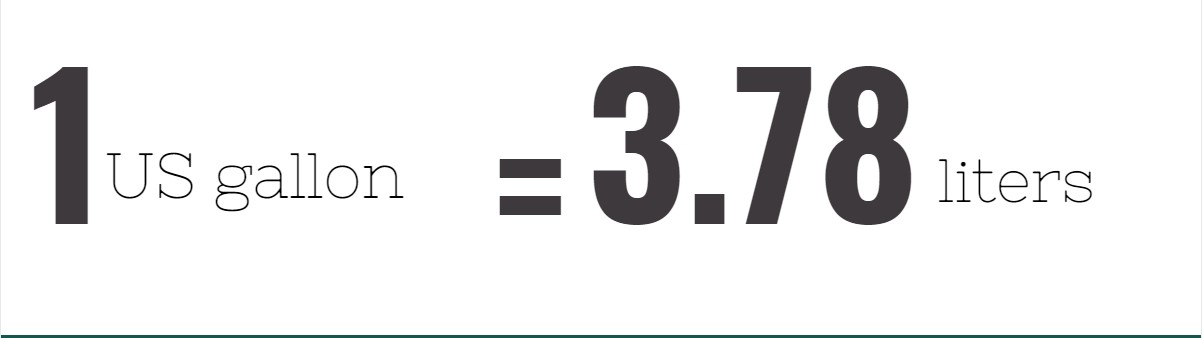# Convert Liters To Gallons (US)

[CP_CALCULATED_FIELDS id=”20″]

## What is liter?

Liter is commonly found in almost all beverages that are sold in large volumes. Expressed by the abbreviation L. In unit conversions, 1 liter is equivalent to 1000 mL or 1 US quart. It is said that one jug of water is exactly equivalent to one liter.

1 liter = 1000 ml = 1 US quart

It is also considered as the most used unit to measure liquids and is also applicable for any dry or solid ingredients which are poured.

1 liter = 0.2641720524 gal (US) 1 cubic decimeter (dm3) = 1000 cubic centimeters (cm3) = 1/1000 cubic meters (m3)

## What is gallon (US)?

Like the cup, gallon is also used as a unit of measurement for volume of both dry and liquid ingredients. Like all other unit conversions too, the gallon is measured in UK, imperial and US conversions. The US gallon when converted to liters would be 3.78.1 US gallon = 3.78 liters

According to Unit Converters, after the British colonial rule in the US, the US gallon was used particularly in measuring wine but evolved later on as a unit measurement for dry ingredients too like grains and corn, among others.

At present, the US gallon is an important unit of measurement in US supermarkets because it is used as the standard measurement for large containers of liquid products such as milk and even gasoline.

1 US gallon = 231 cubic inches = 3.785 liters

## How to convert liters to gallons (US)

L = 0.2641720524 x n gal (US)
n: number of liters

Example: convert 10 liters to gallons (US)
10 l = 10×0.2641720524 =2.641720524 gal (US)